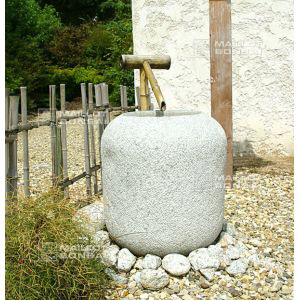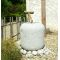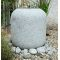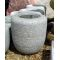##### The Japanese Bonsai specialist
Direct order Contact Help / Services Newsletter# Tsukubai granite basin ø 30 cm

Tsukubairef. : 5920

175,00

voluminous/heavy item extra shipping of 35,00

Available quantity : 3Order

###### Description

Diameter 30 cm. Height 35 cm. Weight + - 80 kilograms. Central hollow Ø +- 15 cm * +- 10 cm deep. A solid granite tsukubai in a pleasingly simple, curved form and with a finely scooped hollow. You can add a bamboo ladle traditionally used for washing hands before entering a temple.

#basin 4.5 #tsukubai 3.8 #granite 3.7 #tsukuba 2.7 #hollow 2.6 #bachi 2.5 #water 2.5 #mizu 2.4 #traditionally 2.3 #entering a 2.1

Formule
(( ROUND((CHAR_LENGTH(b.article_nom)-CHAR_LENGTH(REPLACE(b.article_nom, 'tsukubai', '')))/LENGTH('tsukubai')) + ROUND((CHAR_LENGTH(b.article_description)-CHAR_LENGTH(REPLACE(b.article_description, 'tsukubai', '')))/LENGTH('tsukubai')) ) * 3.8) + (( ROUND((CHAR_LENGTH(b.article_nom)-CHAR_LENGTH(REPLACE(b.article_nom, 'granite', '')))/LENGTH('granite')) + ROUND((CHAR_LENGTH(b.article_description)-CHAR_LENGTH(REPLACE(b.article_description, 'granite', '')))/LENGTH('granite')) ) * 3.7) + (( ROUND((CHAR_LENGTH(b.article_nom)-CHAR_LENGTH(REPLACE(b.article_nom, 'hollow', '')))/LENGTH('hollow')) + ROUND((CHAR_LENGTH(b.article_description)-CHAR_LENGTH(REPLACE(b.article_description, 'hollow', '')))/LENGTH('hollow')) ) * 2.6) + (( ROUND((CHAR_LENGTH(b.article_nom)-CHAR_LENGTH(REPLACE(b.article_nom, 'basin', '')))/LENGTH('basin')) + ROUND((CHAR_LENGTH(b.article_description)-CHAR_LENGTH(REPLACE(b.article_description, 'basin', '')))/LENGTH('basin')) ) * 2.5) + (( ROUND((CHAR_LENGTH(b.article_nom)-CHAR_LENGTH(REPLACE(b.article_nom, 'traditionally', '')))/LENGTH('traditionally')) + ROUND((CHAR_LENGTH(b.article_description)-CHAR_LENGTH(REPLACE(b.article_description, 'traditionally', '')))/LENGTH('traditionally')) ) * 2.3) + (( ROUND((CHAR_LENGTH(b.article_nom)-CHAR_LENGTH(REPLACE(b.article_nom, 'entering a', '')))/LENGTH('entering a')) + ROUND((CHAR_LENGTH(b.article_description)-CHAR_LENGTH(REPLACE(b.article_description, 'entering a', '')))/LENGTH('entering a')) ) * 2.1) + (( ROUND((CHAR_LENGTH(b.article_nom)-CHAR_LENGTH(REPLACE(b.article_nom, 'pleasingly', '')))/LENGTH('pleasingly')) + ROUND((CHAR_LENGTH(b.article_description)-CHAR_LENGTH(REPLACE(b.article_description, 'pleasingly', '')))/LENGTH('pleasingly')) ) * 2) + (( ROUND((CHAR_LENGTH(b.article_nom)-CHAR_LENGTH(REPLACE(b.article_nom, 'kilograms', '')))/LENGTH('kilograms')) + ROUND((CHAR_LENGTH(b.article_description)-CHAR_LENGTH(REPLACE(b.article_description, 'kilograms', '')))/LENGTH('kilograms')) ) * 1.9) + (( ROUND((CHAR_LENGTH(b.article_nom)-CHAR_LENGTH(REPLACE(b.article_nom, 'diameter', '')))/LENGTH('diameter')) + ROUND((CHAR_LENGTH(b.article_description)-CHAR_LENGTH(REPLACE(b.article_description, 'diameter', '')))/LENGTH('diameter')) ) * 1.8) + (( ROUND((CHAR_LENGTH(b.article_nom)-CHAR_LENGTH(REPLACE(b.article_nom, 'central', '')))/LENGTH('central')) + ROUND((CHAR_LENGTH(b.article_description)-CHAR_LENGTH(REPLACE(b.article_description, 'central', '')))/LENGTH('central')) ) * 1.7)

## Secure payment## Delivery

Our logistic partners :04 74 55 23 48
Pépinière MAILLOT-BONSAÏ
Le Bois Frazy
01990 RELEVANT - FRANCE
on appointment